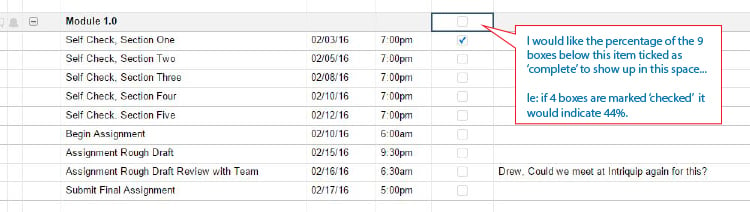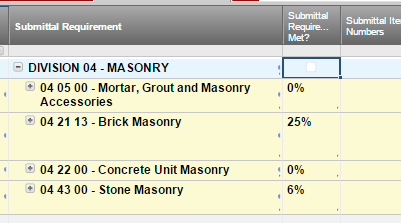#### Welcome to the Smartsheet Forum Archives

The posts in this forum are no longer monitored for accuracy and their content may no longer be current. If there's a discussion here that interests you and you'd like to find (or create) a more current version, please Visit the Current Forums.

# Create percentage complete based of boxes checked

Hello,

I am very new to smartsheet and still quite inept.

I have a smartsheet that I am using to track a student I am mentoring.

There is a coumn titled 'Completed'.  This allows the student to check when a specific task is complete.

I want to be able to show the percentage complete based off how many boxes are checked as completed.

I've attached a screen grab with some notes.

I've tried all morning to make this happen and just getting errors so far.

Thanks - R• Unfortinaltey, ths isn't possible right now (and I so do wish Smartsheet can add this as a possible functionality later on...

Alternate Solution:

Have another column that will calculate the percentage

and if the percentage is 100%, you can checkbox the parent row

Assume Col2 is the Checkbox column and Col 3 is the percentage column

In the Parent row, Col2's equation is:

=IF([Column3]1 = 1, 1, 0)

In the Parent row, Col3's equation is:

=IF(COUNTIF(CHILDREN([Column2]1), 1) / COUNT(CHILDREN([Column2]1)) > 0, COUNTIF(CHILDREN([Column2]1), 1) / COUNT(CHILDREN([Column2]1)), 0)

Good Luck...

• Be aware that if Dependencies are turned on, formulas are removed from the % Complete column associated with dependencies.

Craig

• I think what R was asking is to have the percent complete value added to the parent row checkbox cell. Correct, R?

This is possible! Try this formula:

=ROUND(IF(COUNTIF(CHILDREN(), 1) / COUNT(CHILDREN()) > 0, COUNTIF(CHILDREN(), 1) / COUNT(CHILDREN()), 0) * 100, 0) + "%"

The checkbox field is looking for a 1 or 0 formula result. In my formula we convert the result to a text value, which will display in the checkbox field.

• Bobby,

I've been utilizing the forumla provided for some tracking sheets but I came across a situation where I want to take the percentage (calculated by checked boxes, using your formula) and roll that up into a overall percentage complete (which is the parent of the rows with your formula).  Is there a formula to do this?This discussion has been closed.